# C++ Operators: The Complete Guide

0
166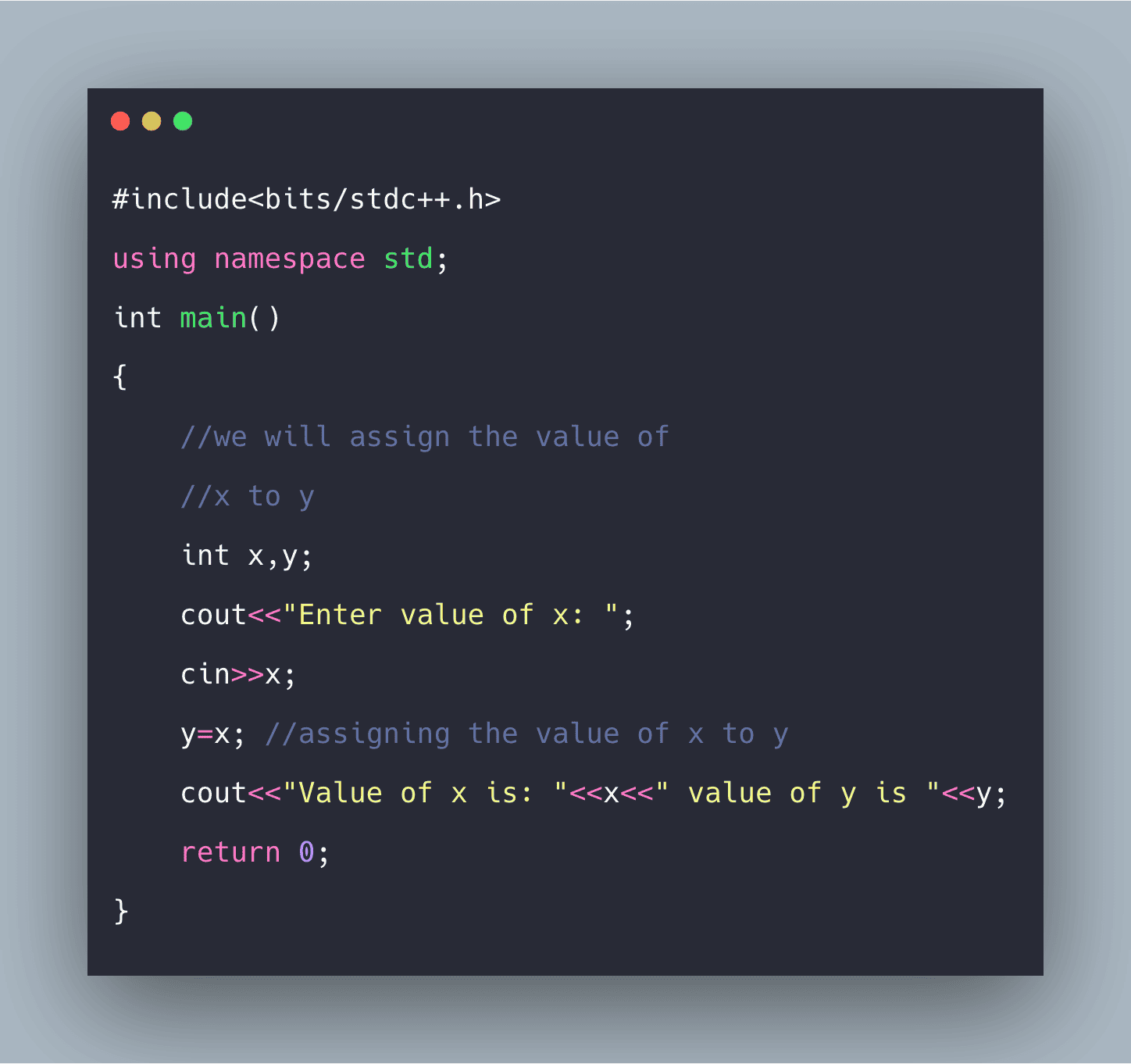C++ Operators are one of the building blocks of any programming language. However, before starting an operation, we first need to learn what Operation is. The Operation is a task to be performed given by the user. Now, this Operation is performed using…

1. Operand: An object (variable) that is manipulated
2. Operator: A symbol used to perform an action/task with those operands.

## C++ Operators

Operators in C++ are one of the building blocks of any programming language. C++ operator is the symbol used to perform mathematical or logical manipulations. C++ language is rich with built-in operators.

Operators are a unique function that takes one or more arguments and produces a new value. Thus, the C++ programming language’s functionality is incomplete without operators’ use.

For example : addition (+), subtraction (-), multiplication (*) etc, are all operators. Operators are used to performing various operations on variables and constants. See the following figure for C++ Operators.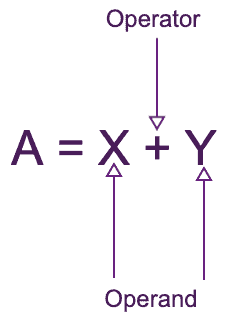In the above figure, A, X, and Y are Operand, and + and = are operators.

The addition operator tells the compiler to add both operands’ X‘ and ‘Y.’

## Different Types of Operators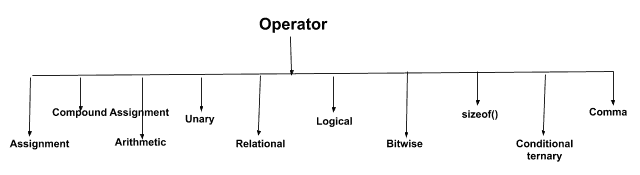## Assignment Operator (=)

An assignment operator in C++ is used to assign a value to a variable.

The symbol of this operator is ‘=.’

For Example,  int a=10;

Here, 10 will be assigned to the int type variable a.

Please note that the value we want to assign will be on the right side of ‘=’, and the variable we want to assign will be on the left side of ‘=.’

Example 2: x = y;

Here, the y value will be assigned to x, and later if we change the value of y, it will not affect x.

We can assign a value to multiple variables at the same time also.

x = y = z = 89

See the following code example.

```#include<bits/stdc++.h>
using namespace std;
int main()
{
//we will assign the value of
//x to y
int x,y;
cout<<"Enter value of x: ";
cin>>x;
y=x; //assigning the value of x to y
cout<<"Value of x is: "<<x<<" value of y is "<<y;
return 0;
}
```

See the output below.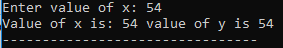### Compound Assignment Operator

Compound Assignment operators are also used to assign values quickly.

For example: number1 += y

Here, the above statement is equivalent to number1 = number1 + y

So, why do we need this? We can see that, and we will have to write the large variable name twice if we write it without the compound assignment operator, which is time-consuming.

But, using a Compound Assignment operator, we can write it in short.

There are few Compound Operators, and we will see some of those.

 Expression Description a+=b a=a+b a-=b a=a-b a^=b a=a^b sum+=x*y sum=sum+(x*y)

See the below code example of the Compound Assignment Operator in C++.

```#include<bits/stdc++.h>
using namespace std;
int main()
{
int x;
cout<<"Enter value of x: ";
cin>>x;
x+=10; //x=x+10
cout<<"New value of x: "<<x<<"\n";
x*=2; //x=x*2
cout<<"New value is: "<<x<<"\n";
return 0;
}
```

See the output below.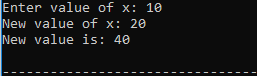## Arithmetic Operator

An arithmetic Operator is used to perform a mathematical operation. For example, there is 5 arithmetic operator in C++; they are the following.

 Operator Description + Used to perform the addition of two or more two numbers. – Used to perform subtraction of two numbers. * Used to perform multiplication. / Used to perform division % Used to find modulo (remainder) of two numbers.

See the below Arithmetic operator in the C++ example.

```#include<bits/stdc++.h>
using namespace std;
int main()
{
int x,y;
cout<<"Enter value of x: ";
cin>>x;
cout<<"Enter value of y: ";
cin>>y;
cout<<"Addition of x and y is: "<<x+y<<"\n";
cout<<"Subtraction of x and y is: "<<x-y<<"\n";
cout<<"Modulo of x and y is: "<<x%y;
return 0;
}
```

See the output.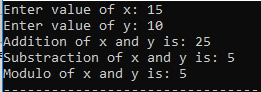## C++ Unary Operator

There are two unary operators in C++. One is for increment (++), and another is for decrement (–).

1. ++: This means the operand’s value will be incremented by 1.
2. –: This implies the operand’s value will be decremented by 1. If these operators are written before the variable (++X), it will first. Increment the value then will perform any operation. Still, if this operator is placed after the variable (X++), it will perform the Operation (if any) and then increment the value.

See the example of the Unary Operator in C++.

```#include<bits/stdc++.h>
using namespace std;
int main()
{
int x,y;
cout<<"Enter value of x: ";
cin>>x;
cout<<"Enter value of y: ";
cin>>y;
//the value of x will show same
//but it will be incremented
cout<<"After increment the value of x is: "<<x++<<"\n";
cout<<"After decrement the value of y is: "<<--y<<"\n";
cout<<"Now the value of x is: "<<x;
return 0;
}
```

See the following output.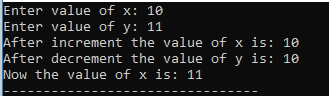## C++ Relational Operator

Relational operators are used to compare two expressions, like if they are equal, greater than, less than, etc. For example, the relational operators in C++ are the following.

 Operator Description == Is equal to != Is not equal to (! represents not always) > Is greater than < Is less than >= Is greater than or equal to <= Is less than or equal to

Note: ‘=’ and ‘==’ are not the same. ‘=’ to assign value, and ‘==’ is to compare the values.

These operators establish the relationship between operands. The relational operators are less than (<), greater than (>), less than or equal to (<=), greater than equal to (>=), equivalent (==), and not equivalent (!=).

You must notice that the assignment operator is (=), and there is the relational operator for equivalent (==).

These two are different; the assignment operator assigns the value to any variable, whereas an equivalent operator is used to compare values, like in if-else conditions.

See the following code example of a Relational Operator in C++.

```#include<bits/stdc++.h>
using namespace std;
int main()
{
int x,y;
cout<<"Enter value of x: ";
cin>>x;
cout<<"Enter value of y: ";
cin>>y;
if(x==y)
{
cout<<"X is equal to Y\n";
}
else if (x>y)
cout<<"X is greater than or Y\n";
else if (x<y)
cout<<"X is less than Y\n";
return 0;
}
```

See the below output.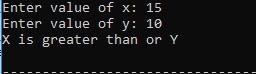## C++ Logical Operator

Logical operators perform boolean operations (AND, OR, and NOT). If two statements are connected using an AND operator, then the validity of both statements will be considered, but if they are connected using an OR operator, either one must be the valid operator.

These operators are primarily used in loops (especially while loop) and Decision making. We will learn in detail.

 Operator Explanation ! Performs boolean NOT Operation. It returns TRUE if the operand is False and FALSE if it is True. && Performs boolean AND Operation. If both the operands are true, it returns TRUE. Otherwise, it returns False. || Performs boolean OR Operation. If both the operands are, then only it returns False. Otherwise, it returns True.

See the following code example of Logical Operator in C++.

```#include<bits/stdc++.h>
using namespace std;
int main()
{
//here we will check if
// x is divided by 10 or
//y is divided by 5
//it will return true
int x,y;
cout<<"Enter value of x: ";
cin>>x;
cout<<"Enter value of y: ";
cin>>y;

// logical OR operator is used here
if(x%10==0 || y%5==0)
{
cout<<"True\n";
}
return 0;
}
```

See the following output.## C++ Bitwise Operator

The bitwise operator is used to perform bit-level operations on the operands. The operators are first converted to bit-level, and then the calculation is performed on the operands.

Bitwise operators are:

 Operator Explanation & It is used to perform bitwise AND Operation. | It is used to perform bitwise OR Operation. ^ It is used to perform bitwise XOR operation ~ It is used for unary complement (bit inversion) << It is used for binary Left Shift Operation. >> It is used for binary Right Shift Operation.

See the following code example of Bitwise Operator in C++.

```#include<bits/stdc++.h>
using namespace std;
int main()
{
int x,y;
cout<<"Enter value of x: ";
cin>>x;
cout<<"Enter value of y: ";
cin>>y;
cout<<"Bitwise AND of X and Y is: "<<(x&y)<<"\n";
cout<<"Bitwise OR of X and Y is: "<<(x|y)<<"\n";
cout<<"Shift Right of X: "<<(x>>1)<<"\n";
return 0;
}
```

See the following output.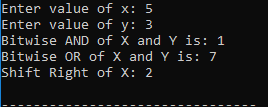### C++ Shift Operators

Shift Operators are used to shift Bits of any variable. It is of three types,

1. Left Shift Operator <<
2. Right Shift Operator >>
3. Unsigned Right Shift Operator >>>>

## C++ sizeof(type/variable) Operator

The sizeof() operator is used to find the size of a type or a variable. It returns the size in bytes. It accepts one parameter as either the variable type or a variable.

Example: s=sizeof(char)

This will return 1, as the size of the char is 1 byte.

## C++ Conditional ternary operator

The conditional operator is used to get the result of one condition.

The syntax is: condition? result1: result2

Here, if the condition is true, it will return result1; otherwise, it will return result2. It is similar to the if-else statement.

See the following code example of Conditional Operator in C++ Example.

```#include<bits/stdc++.h>
using namespace std;
int main()
{
int x,y;
cout<<"Enter value of x: ";
cin>>x;
cout<<"Enter value of y: ";
cin>>y;
x>y?cout<<"Greater":cout<<"Smaller";
return 0;
}
```

See the following output.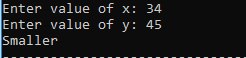## C++ Comma Operator (,)

The comma operator (,) is used to separate the two or more expressions included where only one expression is expected. It returns s the rightmost expression when there is multiple expression.

For Example: x=(m=10, m-5);

Here, the result will be 5 because it will first assign the value of m to 10, then subtract 5 from 10 (10-5), which results in 5, and then 5 will be assigned to x.

See the following code example of Comma Operator in C++.

```#include<bits/stdc++.h>
using namespace std;
int main()
{
int x,m;
x=(m=10,m+7);
cout<<"Value of x is: "<<x;
return 0;
}
```

See the output below.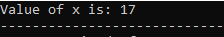That’s it for this tutorial.

This site uses Akismet to reduce spam. Learn how your comment data is processed.